A 75-g projectile traveling at 600 m /s strikes and becomes embedded in the 40-kg block, which is ini-tially stationary. Compute

Question

A 75-g projectile traveling at 600 m /s strikes and becomes embedded in the 40-kg block, which is ini-tially stationary. Compute the energy lost during the impact. Express your answer as an absolute value ΔE| and as a percentage n of the original system energy E.

in progress 0
5 months 2021-08-28T08:55:56+00:00 2 Answers 1 views 0

1. Explanation:

The given data is as follows.

Mass, m = 75 g

Velocity, v = 600 m/s

As no external force is acting on the system in the horizontal line of motion. So, the equation will be as follows.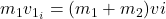where,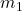= mass of the projectile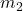= mass of block

v = velocity after the impact

Now, putting the given values into the above formula as follows.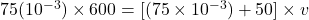=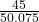v = 0.898 m/s

Now, equation for energy is as follows.

E =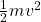=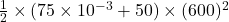= 13500 J

Now, energy after the impact will be as follows.

E’ =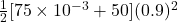= 20.19 J

Therefore, energy lost will be calculated as follows.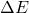= E  E’

= (13500 – 20) J

= 13480 J

And,   n =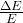=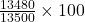= 99.85

= 99.9%

Thus, we can conclude that percentage n of the original system energy E is 99.9%.

Explanation:

Explanation:

m1 = 75 g = 0.075 kg

m2 = 40 kg

u1 = 600 m/s

u2 = 0

Let the velocity of combined mass is v.

Use conservation of momentum

m1 x u1 + m2 x u2 = ( m1 + m2) x v

0.075 x 600 + 0 = ( 40 + 0.075) x v

v = 1.12 m/s

Initial energy, E = 0.5 x 0.075 x 600 x 600 = 13500 J

Final energy, E’ = 0.5 x 40.075 x 1.12 x 1.12 = 25.14 J

Loss in energy, ΔE = E – E’ = 13500 – 25.14 = 13474.86 J

% loss of energy = ( 13474.86 x 100) / 13500 = 99.8 %